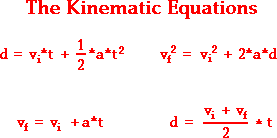# Questions on Kinematics

Im making a new thread because I coulnt edit my previous thread title

1. Homework Statement
1. While descending at a constant speed of 1.0 m/s, a scuba diver releases a cork, which
accelerates upward at 3.0 m/s2. What is the diver’s depth when the cork reaches the surface 2.0 s later?

2. A car with a velocity of 27 m/s slows down at a rate of - 8.5 m/s2 to a stop in a distance
of 43 m on a dry road. The same car traveling at 27 m/s slows down at a rate of -6.5 m/s2 to a stop on a wet road.
a. How much farther does the car travel on the wet road before coming to a stop?
b. What maximum car speed will allow the car traveling on the wet road to stop in a distance of 43 m?

3. A car traveling at 14 m/s encounters a patch of ice and takes 5.0 s to stop.
a. What is the car’s acceleration?
b. How far does it travel before stopping?

4. An accelerating lab cart passes through two photo gate timers 3.0 m apart in 4.2 s. The velocity of the cart at the second timer is 1.2 m/s.
a. What is the cart’s velocity at the first gate?
b. What is the acceleration?

2. Homework Equations
WE NEED TO USE ONE OF THESE EQUATIONS FOR THESE QUESTIONS
V= Vo + at
d = Vo t + ½ at2
V2 = Vo2 +2ad
d = ½ (V + Vo) t

g= -9.8 m/s2

3. The Attempt at a Solution

I am sorry. I really have no idea. I have been sick for quite some time and missed a lot of school time. Since we are on break, this was our assignment, I cannot ask the teacher for help.

If you can tell me which equation I need to use, I am sure that will help.

## Answers and Replies

A good way to start any kinematic problem is to write down all the given quantities, and then use an equation that has all the known quantities except one.

Let's use #3 as an example:

we know $$\Delta$$t = 5 seconds, $$V_{1}$$ = 14m/s, and since the car needs to stop, it's $$V_{2}$$ would 0

Last edited:
I solved 1-3; I am only in need of help on number 4.

Luckily I had a question very similiar to that on my test. Here it goes

4. An accelerating lab cart passes through two photo gate timers 3.0 m apart in 4.2 s. The velocity of the cart at the second timer is 1.2 m/s.
a. What is the cart’s velocity at the first gate?
b. What is the acceleration?

In this case, we only need to worry about what happened between the 2 gate timers, everything before/after we can ignore.

So we know the distance it travelled, d = 3.0m, we know how long it took it to travell 3.0 m, t = 4.2 seconds, and we know it's velocity at the second timer $$V_{2}$$ = 1.2 m/s. Now we need to find an equation to relate these 3 known variables.

Another way you can do this, is do part b) first, and then a)

what did u get to find the accleration

My mistake, I think it would be better if you did part a) first and then b). Once you do that, post your work here.

I have no idea what to do..

I cant even properly set up the equation

V= Vo + at
d = Vo t + ½ at2
V2 = Vo2 +2ad
d = ½ (V + Vo) t

which of these 4 equations has 3 of the known quantities, and 1 unknown quantity that you need?

the 4th( i think) but when I set it up.. things didnt work out too well

I think the 4th is right. Let me give a try (hope I'm not wrong)

d = ½*(V + Vo)*t

3 = ½*(1.2 + Vo)*4.2
6 = (1.2 + Vo)*4.2
1.2 + Vo = 6 / 4.2
Vo ~ 1.43 m/s

yea... I see what you mean. It's initial velocity is supposed to be smaller than it's velocity at the second gate...

Last edited:
so it is w4ong?

I'm an idiot

3 = ½*(1.2 + Vo)*4.2
6 = (1.2 + Vo)*4.2
1.2 + Vo = 6 / 4.2
Vo = 1.43 - 1.2 = 0.23 m/s

that sounds more possible because the cart is accelarating so it's supposed to gain speed.

i'll try that

im not following your work

I m lost in it

Last edited:
where did u get 6 from?

3 = ½*(1.2 + Vo)*4.2
6 = (1.2 + Vo)*4.2

I needed to get rid of the ½ and so I multiplyed both sides of the equation by 2

why would u do that....

to isolate Vo

Here's an example:

you have the following equation

½X = 3

Isolate X so we would multiply both sides by 2 to isolate X so we get X = 6

2 * ½ = 1

i got .48m/s

it would help if you posted your workI used the bottom right equation

and took it from there

3= 1.2/2 (4.2)

3-2.52=.48

you can't do that. If you look at that equation, the fraction 1/2 only effects Vi and Vf, not t.

think of it like this:

($$\frac{Vf+Vi}{2}$$)*t

as you can see, t is outside the bracket and hence isnt effected by the 1/2. If you wanted it inside the bracket, you would need to divide t by 2 as well, but that's just extra work you don't need to do